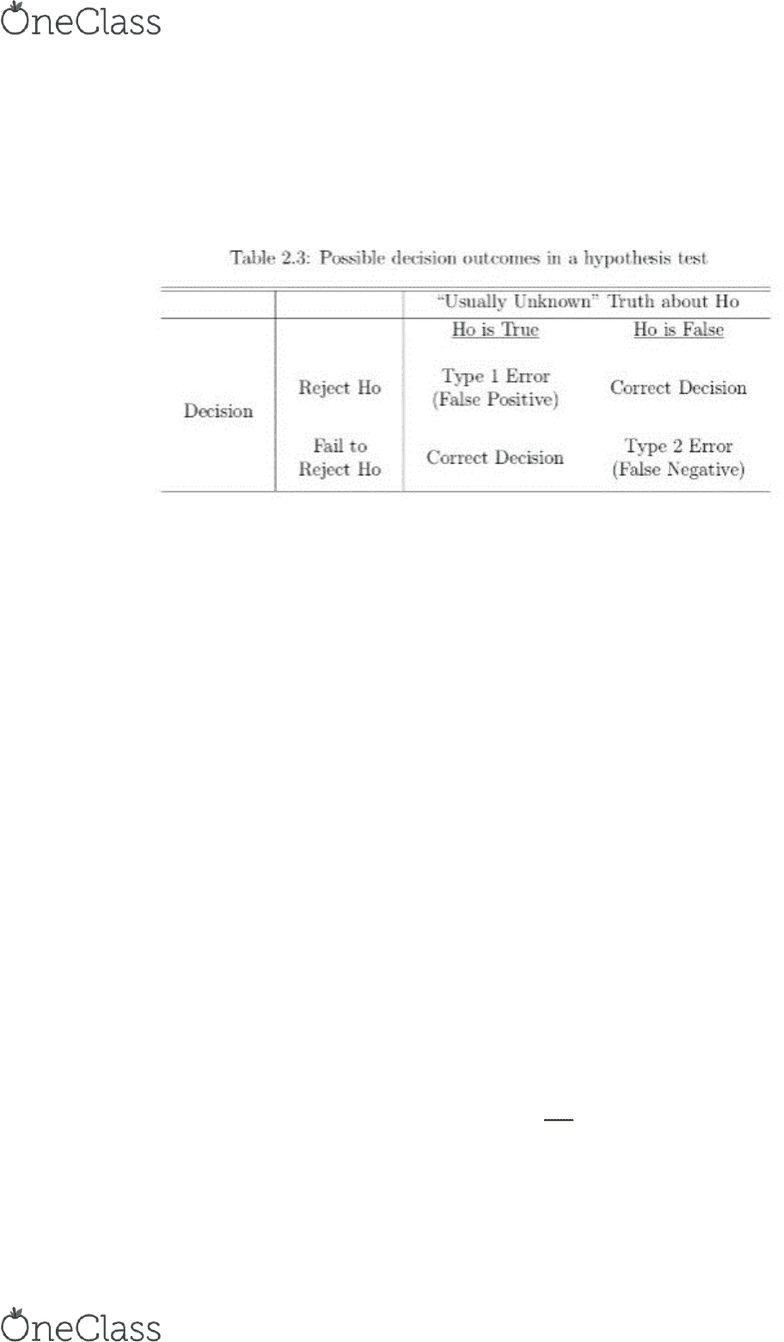Textbook Notes (270,000)
US (100,000)
GVSU (400)
STA (6)
Chapter 2.14, 3.1, 3.2, 3.4, 5.1

STA 215 Chapter Notes - Chapter 2.14, 3.1, 3.2, 3.4, 5.1: Quartile, Box Plot, Standard Deviation

Department
Statistics
Course Code
STA 215
Professor
Gabrosek
Chapter
2.14, 3.1, 3.2, 3.4, 5.1

This preview shows page 1. to view the full 5 pages of the document.STA 215
10/25/17
Section 2.14 False Positives and False Negatives
Definitions:
o Type 1 error/ false positive- When a HT leads us to reject Ho even though Ho is
true.
o Type 2 error/ false negative- When a HT leads us to reject Ho even though Ho is
false.
o Level of significance- Is the probability of a type 1 error in a HT.
o Power of a HT- The probability that a random sample will result in a HT that
correctly rejects the null hypothesis when it is false.
Section 3.1 The Five-Number Summary and Percentiles
Definition: Percentile- “The pth percentile of a data set is a value such that at least p% of
the data values are less than or equal to the pth percentile and at least (1-p)% of the data
values are greater than or equal to the pth percentile. We can think of the pth percentile as
splitting the ordered data set in two chunks- One with p% of the data and the other with
(1-p)5 of the data. Example notation for the 38th percentile is P38.”
Definition: Five-Number Summary- This is the min, the first quartile (Q1), the median,
the third quartile (Q3), and the max
Definition: Minimum- Smallest value in the data set. This is P0, the 0th percentile because
no data is less than this
Definition: Maximum- Largest value in the data set. This is P100, the 100th percentile
because no data is greater than this
Definition: Median- This is the value where half of the data set is less than and the other
half is greater than this value. This is P50, the 50th percentile.
o Position of the median can be found by �+
where n is the number of data values
Definition: First quartile- This is the value where 25% of the data is less than this value.
Denotated by P25
find more resources at oneclass.com
find more resources at oneclass.com

Unlock to view full version

Only page 1 are available for preview. Some parts have been intentionally blurred.Definition: Third quartile- This is the value where 75% of the data is less than this value.
Denotated by P75
Section 3.2 Boxplots
When interpreting a graph for quantitative data, we focus on the following:
o Center of distribution
o Variability of the distribution
o Shape of distribution
o Presence of outliers in the distribution
Interpreting center- Think of the center of distribution as the point where the data splits in
half so that half of the values are less than center and half are more. This is a visual
representation where the median falls on a graph.
Interpreting variability- When thinking of variability, think of the following questions;
Are the data values clustered together or more spread out? If the data is dispersed, are the
spread equally around the center indicating large variability? Or are many values near
center with a few extremes indicating less variability? Or are many values at the extremes
indicating large variability?
Interpreting shape- When thinking of shape, think of the following questions; Is the
distribution symmetric, skewed right, skewed left, or none? Distribution unimodal,
bimodal, or neither? Is the distribution bell shaped?
Graph can be symmetrical, skewed left, skewed right, bell shaped, unimodal, or bimodal
o Definition: Unimodal distribution is when the data set has a single peak around
which the frequency declines and does not rise to a second distinct peak
o Definition: Bimodal distribution is when the data set has two distinct peaks
separated by a valley
o Definition: Outlier is a value in the data set that is atypically large or small
A typical boxplot consists of the following
o Number line that starts at or below min and ends at or above max. Amount of
jump on number line between numbers must be equal.
o Rectangular box whose lower/ left edge is at Q1 and upper/right edge is at Q3.
The box should enclose 50% of the data.
o Line drawn inside box at the median.
o Dashed line drawn from lower edge of the box down to min. aka left tail. Dashed
line drawn from upper edge of box up to max. aka right tail.
find more resources at oneclass.com
find more resources at oneclass.com